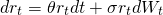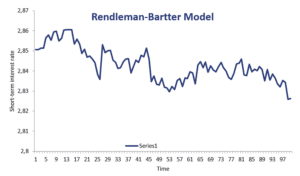# Rendleman-Bartter model

The Rendleman-Bartter model is a short-term interest rate model that was proposed by Richard Rendleman and Brit Bartter in 1980. The model can be used to consider interest rate uncertainty. Like the (more popular) Vasicek model, the Rendleman-Bartter (RB) model is a single factor model as it focuses on a single risk factor, market risk. The model is mainly used to value interest rate derivatives using Monte-Carlo simulation.

On this page, we discuss the Rendleman-Bartter model formula as well as a numerical example. We implement the RB-mode using an example in Excel. The spreadsheet is available for download at the bottom of the page.

## Rendleman-Bartter model formula

The RB-model specifies that the interest rate follows a Brownian motionwhere W is a Wiener process, theta is the drift parameter, and sigma is the volatility of the interest rate.

The Rendleman-Bartter model was one of the earliest short-term interest rate models. We can easily see this from the fact that the model simply uses the same dynamics as the one used to model stock prices. See for example, the Black-Scholes model, which assumes stock price changes follow a Brownian motion.

The main disadvantage of the model is that such behavior does not capture an important stylized fact of short-term interest rates. In particular, the interest rate does not exhibit mean-reversion. More recent models, such as the Cox, Ingersoll, and Ross model and Vasicek model are preferred because they allow for mean-reversion. If no mean reversion is in place, then the short-term interest rate will simple be a random walk with drift.

## Rendleman-Bartter model example

Let’s use the above model to simulate the short-term interest rate. The following figure illustrates a short-term interest rate that was simulated using the model. The spreadsheet used to implement the model can be downloaded at the bottom of the page.As we can see, simple spreadsheet software can be used to simulate short-term interest rates using the BR-model.

## Summary

The RB model should not be confused with another model proposed by both academics. In particular, Rendleman and Bartter also proposed a two-state option pricing model one year earlier, in 1979.